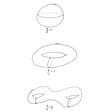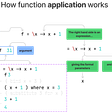# Hyperoperation via F#

When I first learned multiplication, I learned it in terms of addition.

`3 * 3 = the sum of three 3s = 3 + 3 + 3`

Then after exponents settled in, in terms of multiplication, I thought “hmmm, if 3*3 = 3+3+3 and 3³ = 3*3*3, what’s next?”. At the time, I envisioned “super-exponents”, 3superEx3= 3 ^ 3 ^ 3, but left it at that.

Years passed.

On a recent flight, I pulled up the FSharp-Interactive REPL to try to model this recursion. Let’s start by defining addition; for now we’ll cheat and use the built-in + operator.

`let add x y =    x + y`

Well that was easy. Now we want to define multiplication in terms of addition as described above.

`//e.g. mult 3 3 = 3 + 3 + 3let mult x n =    //initialize an array of 3's of length 3    let seed = Array.init n (fun i -> x)    //add each 3 to the result, start with 0    seed |> Array.fold add 0> mult 3 3;;val it : int = 9`

And on to exponentiation, which is defined in terms of multiplication, which is defined in terms of addition.

3³ = 3 * 3 * 3 = (3 + 3 + 3) + (3 + 3 + 3) + (3 + 3 + 3) = 27

`let exp x n =     //e.g. initialize an array of 3s of length 3       let seed = Array.init n (fun i -> x)      //mult each 3 to the result, start with 1      seed |> Array.fold mult 1> exp 3 3;;val it : int = 27`

Note that if we dial this up a bit and try larger input we’ll blow out our int.

`> exp 10 10;;val it : int = 1410065408 `

The result should have been 10,000,000,000; to give us a little more room to work, let’s use modify our set of functions to use Int64. While we’re at it let’s switch F# Interactive to 64-bit, which will increase our stack space. In VS you can do this at Tools -> Options -> F# Tools -> F# Interactive -> 64-bit F# Interactive.

So our new exponentiation function is:

`let exp (x:int64) (n:int64) =   let seed = Array.init (int n) (fun i -> x)   Array.fold mult 1L seed> exp 10L 10L;;val it : int64 = 10000000000L`

Well hey now, this looks an awful lot like the mult function, except the fold operator has changed from add to mult, and the starting value has changed from 0 to 1, since 1 is the identity value for multiplication. Let’s hold that thought for a minute while we explore what I was calling “super-exponentiation”, and for which the proper term is tetration.

Tetration:

`3 tet 3= 3 ^ 3 ^ 3 = 3 ^ (27) = (3*3*3)*(3*3*3)*(3*3*3)   + 24 more “*(3*3*3)”s = (((3+3+3)+(3+3+3)+(3+3+3))  +((3+3+3)+(3+3+3)+(3+3+3))  +((3+3+3)+(3+3+3)+(3+3+3)))   + 282,429,536,478 more “((3+3+3)+(3+3+3)+(3+3+3))”s= 7,625,597,484,987 . `

That escalated quickly. Note that exponentiation is right-associative: 3 tet 3= 3^(3 ^ 3), not (3 ^ 3) ^ 3. So we’ll use foldBack here instead of fold. And because we’re starting our fold with x, we can leave one instance of x out of the array; thus we’ll init the array with n-1 x’s.

`//tetrationlet tet x n =   let seed = Array.init (int (n - 1L)) (fun i -> x)   Array.foldBack exp seed xtet 3L 3L;;val it : int64 = 7625597484987L`

OK! Same pattern as before, with the fold function as exp and the start value as x. Upward and onward to pentation:

`let pent x n =    let seed = Array.init (int (n-1L)) (fun i -> x)    seed |> Array.fold tet x> pent 3L 3L;;System.OutOfMemoryException: Exception of type 'System.OutOfMemoryException' was thrown. at FSI_0013.mult(Int64 x, Int64 n) in C:\Users\cdutcher\Documents\Visual Studio 2015\Projects\Scratch\Scratch\Tetration.fsx:line 56 at Microsoft.FSharp.Collections.ArrayModule.Fold[T,TState](FSharpFunc`2 folder, TState state, T[] array) at Microsoft.FSharp.Collections.ArrayModule.FoldBack[T,TState](FSharpFunc`2 folder, T[] array, TState state) at Microsoft.FSharp.Collections.ArrayModule.Fold[T,TState](FSharpFunc`2 folder, TState state, T[] array) at <StartupCode\$FSI_0016>.\$FSI_0016.main@()Stopped due to error`

Well. We’ve exhausted our available memory. Turns out that pentation is extremely powerful. Nonetheless, the pattern is clear, and we can combine all into one generic function that takes an extra “level” parameter, where level 0 is addition, 1 is multiplication, 2 is exponentiation, 3 is tetration, 4 is pentation, and onwards.

`let rec hyper level x n =     let startValue =        function        | 0-> 0.0        | 1-> 1.0        | _ -> x    match level with    | 0 ->         add x n    | _ ->        let seed = Array.init n (fun i -> x)        seed        |> Array.fold (hyper(level-1)) (startValue(level - 1))`

And checking some results:

`> hyper 0 3L 2L;; //additionval it : int64 = 5L> hyper 1 3L 2L;; //multiplicationval it : int64 = 6L> hyper 2 3L 2L;; //exponentiationval it : int64 = 9L> hyper 3 3L 2L;; //tetrationval it : int64 = 19683L  //3 ^ (3 ^ 2)> hyper 3 3L 3L;; //tetration example 2val it : int64 = 7625597484987L // 3 ^ (3 ^ 3)> hyper 4 3L 2L;; //pentationSystem.OutOfMemoryException: Exception of type 'System.OutOfMemoryException' was thrown.at FSI_0002.hyper(Int32 l, Int64 x, Int64 n) in C:\Users\Cole\Documents\GitHub\InContactBridge\InContactBridge\InContactBridge\Tetration.fsx:line 37at Microsoft.FSharp.Collections.ArrayModule.Fold[T,TState](FSharpFunc`2 folder, TState state, T[] array)at Microsoft.FSharp.Collections.ArrayModule.Fold[T,TState](FSharpFunc`2 folder, TState state, T[] array)at <StartupCode\$FSI_0010>.\$FSI_0010.main@()Stopped due to error`

It works!….mostly.

That memory issue really puts a damper on things, but we can refactor a bit to permit tail-recursion and avoid scaling the memory usage.

The nest function takes a function and applies it n-times to its input x.

`let rec nest n f x =    if n=0L then x    else nest (n-1L) f (f x)`

We can define addition in terms of the single-variabled succession function:

`let add1 x =    x + 1L//0let addn x n =    x |> nest n add1`

Working up the levels we have:

`//1let multn x n =    x |> nest (n - 1L) (addn x)//2let expn x n =    x |> nest (n - 1L) (multn x)//3let tet x n =    x |> nest (n - 1L) (expn x)//4let pent x n =    x |> nest (n - 1L) (tet x)let rec hyper level x n =    match level with    | 0L -> addn x n    | _ ->        let f = hyper(level - 1L) x        nest (n - 1L) f x`

The nest function and our hyper function are very similar; the latter just defines some base cases. If we modify hyper to cover all base cases, including the succession function we have:

`let rec hyper level a b =    match level, b with    | 0L, _ -> b + 1L    | 1L, 0L -> a    | 2L, 0L -> 0L    | _ , 0L -> 1L    | _ ->        hyper (level - 1L) a (hyper level a (b - 1L))> hyper 4L 3L 3L;;val it : int = 7625597484987L`

This is the proper hyperoperation function. While we’ve avoided the memory problems, this function is still extremely inefficient. Some resulting values for tetration (level=4 in our new succesion-zeroed function) are in the table below, and we know that our function ultimately starts at 0 and counts by 1 up to the result, so it should take a while for a,b> 3.

This tremendous growth actually makes hyperoperation very special. The function is total recursive, which, in short, means that it will definitely complete (eventually), but it is not primitive recursive. If it were primitive recursive, it could be written with a bounded for-loop, where the bound is set in advance. While other functions exist which are known to be total recursive, but not primitive recursive, they are generally derived from or closely related to the hyperoperation function. The most popular of these is Ackermann’s function:

`//simplelet rec ackermann m n =     match m, n with    | 0, n -> n + 1    | m, 0 -> ackermann (m - 1) 1    | m, n -> ackermann (m - 1) ackermann m (n - 1)//using tail-recursionlet ackermann M N =    let rec acker (m, n, k) =       match m,n with        | 0, n -> k(n + 1)        | m, 0 -> acker ((m - 1), 1, k)        | m, n ->             acker (m, (n - 1), (fun x -> acker ((m - 1), x, k)))    acker (M, N, (fun x -> x))//source: http://rosettacode.org/wiki/Ackermann_function`
`A(m,n) = hyper(m,2,n+3)-3.`

The tremendous growth in necessary recursive steps of Ackermann’s function, for increasing values of m and n, makes it very useful for testing the efficiency of a compiler’s code generation for function calls. See here and here.

I’ll sign off here, but if you’re interested in digging further check out:

Notation Systems for Hyperoperations: Knuth Up-Arrow Notation, Conway Chains

Tetration over Complex Numbers -> Extension to complex bases

Graham’s Number (<- this post is really great, as are many from WaitButWhy)

--

--

--

## More from coledutcher

F#, startups, and other thoughts

## Accelerating variational quantum algorithms## Computational Geometry — Riemann-Roch Theorem and Holomorphic 1-forms## 1 at 100 But How much for 1200 😱 (EXCEL)## The Intuition behind the Central Limit Theorem## Zobrist Hashing## A Physical Theory based on Sets, Not Vectors: Part 1## Monte Carlo simulations for option pricing## Accelerate quantum computation from a classical approach## Cole Dutcher

Engineering Manager @ Jet.com/WalmartLabs, Functional Programming Enthusiast, Cofounder of codefordemocracy.io

## The Generalist Argument: On Physics and Programming with types## Rediscovering Mathematics## Webservices -C# 👩🏻‍💻